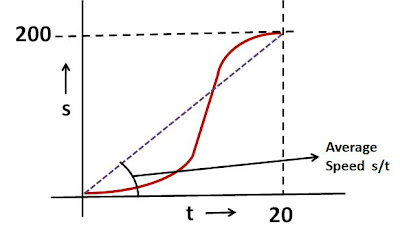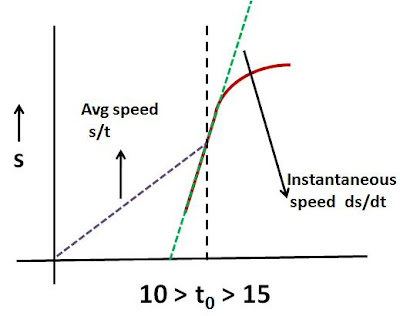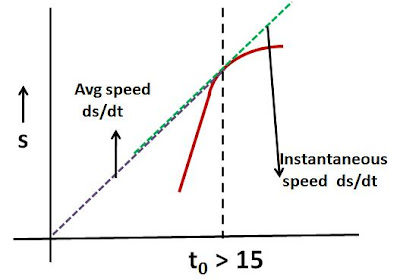Thursday, July 12, 2007

Irodov Problem 1.4a) The average velocity is distance traveled by time = 200cm/20sec = 10cm/secb)
The maximum velocity is the point where the rate of change of distance i.e. the slope of the curve is the maximum. In the mid portion of the curve, the point moves 100cm in 4 seconds. Thus, the slope is 100/4 = 25cm/sec - this is the maximum speed achieved.

c) At any time the instataneous speed (ds/dt) is the slope of the curve while the average speed (s/t) is the tangent of the angle of the line joining the origin to the point.Let us first consider a time t0 during the acceleration phase of the point. This is when t0 is in the interval (0,10). Throughout this region, the slope of the curve will always be greater than that of the line joining the point to the origin since the curve is convex. This means that throughout the acceleration phase, s/t will always be less than ds/dt.

Now lets us consider the region where, the speed is constant. As shown in the picture even in this region the tangent of the line joining the point to the can never catch up the slope of the curve.However, when we consider a time that is in the decelerating region of the curve, as the instantaneous speed of point decreases, at some time the average speed will catch up with the instantaneous speed. In other words the tangent of angle of line connecting the point to the origin will be same as the slope of the curve. This is shown in the figure beside.Since exact nature (equation) of the curve of the decelerating part of the curve is not provided (it could be exponentially decaying) it is not possible to mathematically determine the exact value of time at which this happens. However, geometrically one can draw a tangent at every point on the curve and see if it passes through the origin. When this happens it will be the point at which instantaneous speed is same as the average speed.

Some readers have commented that this probably happens at 16s as suggested in the answer. Certainly it seems that way but the exact answer cannot be determined mathematically unless the nature iof the curve is specified.Anonymous said...

Third part of the problem

Average velocity = slope of line joining point to the origin (since v_avg = s/t)
Instantaneous velocity = slope of the tangent to the curve at that point (since v_ins = ds / dt)
Only place where this happens is in the deceleration phase, as you pointed out.
Looking at the plot, t = 16s is the right place (at least to my eyes :-).

Krishna Kant Chintalapudi said...

Thanks for the comment, I think the solution needed more explanation. I have updated the solution and hopefully its clear now why I am saying the exact answer cannot be found unless the nature of the deceleration curve is known.Anonymous said...

i support u krishna.how can we know at at exactly 16secs the tangent will pass through origin unless ,we don't know the the equation of the curve how can we determine the equation of the tangent at t=16 and say that the origin satisfies it?

sayantanpsychicdon said...

so is there any way to find the equation of the curve??